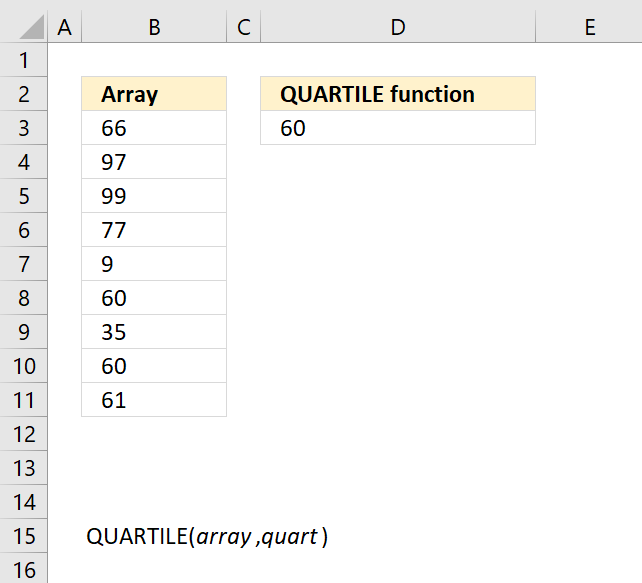Author: Oscar Cronquist Article last updated on November 13, 2018The QUARTILE function returns the quartile of a data set, use the QUARTILE function to divide data into groups.

This function is outdated and has been replaced with the newer QUARTILE.EXC and QUARTILE.INC function.

Formula in cell D3:

=QUARTILE(B3:B11,1)

Excel Function Syntax

QUARTILE(array, quart)

Arguments

 array Required.The cell values for which you want to calculate the quartile value. quart Required. Indicates which value to return, see table below.

Quart parameters
0 Minimum value.
1 First quartile (25th percentile).
2 Median quartile (50th percentile).
3 Third quartile (75th percentile).
4 Maximum value.